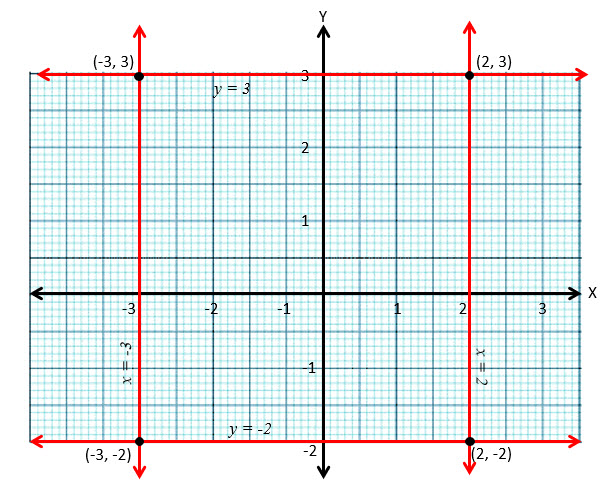Question 1: Find the equation of the line parallel to x-axis and passing through$(3, 5)$

Equation of a line parallel to x-axis is$y = k$

The line passes through$( 3, -5)$$\therefore k = -5$

Hence the equation of the line is$y + 5 = 0 \text{ or } y = - 5$$\\$

Question 2: Find the equation of the line perpendicular to x-axis and having intercept$- 2$ on x-axis.

Equation of  a like perpendicular to x-axis is$x = k$

The line passes through$(-2, 0)$$\therefore k = -2$

Hence the equation of line is$x + 2 = 0 \text{ or } x=-2$$\\$

Question 3: Find the equation of the line parallel to x-axis and having intercept$-2$ on y-axis.

Equation of a line parallel to x-axis is$y = k$

The line passes through$( 0, -2)$$\therefore k = -2$

Hence the equation of the line is$y + 2 = 0 \text{ or } y = -2$$\\$

Question 4: Draw the lines$x=-3, x=2, y = -2, y =3$ and write the coordinates of the vertices of the square so formed.

Answer:$\\$

Question 5: Find the equations of the straight lines which pass through$(4, 3)$ and are respectively parallel and perpendicular to the x-axis.

i)        Equation of a line parallel to x-axis is$y = k$

The line passes through$( 4, 3)$$\therefore k = 3$

Hence the equation of the line is$y -3 = 0 \text{ or } y = 3$

ii)      Equation of  a like perpendicular to x-axis is$x = k$

The line passes through$(4,3)$$\therefore k = 4$

Hence the equation of line is$x -4 = 0 \text{ or } x = 4$$\\$

Question 6: Find the equation of a line which is equidistant from the lines$x = - 2$ and$x = 6$.

Line equidistant from the lines$x = - 2$ and$x = 6$ will pass through the mid point of ( -2, 0) and ( 6, 0) which is (2,0)

Equation of  a like parallel to y-axis is$x = k$

The line passes through$(2,0)$$\therefore k = 2$

Hence the equation of line is$x - 2 = 0 \text{ or } x=2$$\\$

Question 7: Find the equation of a line equidistant from the lines$y = 10$ and$y = -2$.

Line equidistant from the lines$y = 10$ and$y = -2$ will pass through the mid point of$( 0,10)$ and$( 0, -2)$ which is$(0, 4)$
Equation of a line parallel to x-axis is$y = k$
The line passes through$( 0,4)$$\therefore k = 4$
Hence the equation of the line is$y -4 = 0 \text{ or } y = 4$